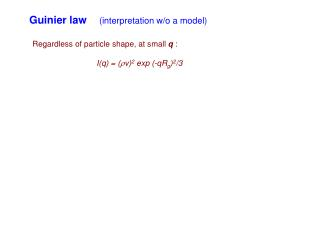# Guinier law (interpretation w/o a model) - PowerPoint PPT PresentationDownload PresentationGuinier law (interpretation w/o a model)

Download Presentation## Guinier law (interpretation w/o a model)

- - - - - - - - - - - - - - - - - - - - - - - - - - - E N D - - - - - - - - - - - - - - - - - - - - - - - - - - -
##### Presentation Transcript

1. Guinier law (interpretation w/o a model) Regardless of particle shape, at small q : I(q) = (v)2 exp (-qRg)2/3

2. Guinier law (interpretation w/o a model) Regardless of particle shape, at small q : I(q) = (v)2 exp (-qRg)2/3 ln (q) = ln (v)2 - (Rg2/3) q2

3. Guinier law (interpretation w/o a model) Regardless of particle shape, at small q : I(q) = (v)2 exp (-qRg)2/3 ln (q) = ln (v)2 - (Rg2/3) q2

4. Guinier law (interpretation w/o a model) Regardless of particle shape, at small q : I(q) = (v)2 exp (-qRg)2/3 ln (q) = ln (v)2 - (Rg2/3) q2 Only holds if: a. q < 1/Rg b. dilute c. Isotropic d. matrix or solvent scattering is removed

5. Guinier law (outline of derivation) (r) is scattering length distribution A(q)= ∫(r) exp (-iqr) dr Expand as a power series: A(q)= ∫(r) dr - i∫qr (r) dr - (1/2!)∫(qr)2(r) dr + … Origin at center of mass

6. Guinier law (outline of derivation) (r) is scattering length distribution A(q)= ∫(r) exp (-iqr) dr Expand as a power series: A(q)= ∫(r) dr - i∫qr (r) dr - (1/2!)∫(qr)2(r) dr + … Origin at center of mass (qr)2 = (qxx + qyy + qzz)2 xy = (1/ v)∫xy(r) dr , etc…… v 0

7. Guinier law (outline of derivation) q << v; average intensity/particle, for large # randomly oriented particles: I(q) = (v)2(1- ((qxx)2 + (qyy)2 + (qzz)2 + 2qxqyxy) Isotropic: average x2 = average y2 = average z2 = Rg2/3 average xy= average yz= average zx= 0 I(q) = (v)2(1- ((q Rg)2 /3+ …) I(q) = (v)2 exp (-qRg)2/3

8. Guinier law (outline of derivation) For non-identical particles, Guinier law gives an average R& average v

9. Model structures - effect of dense packing • For N spherical particles, radius R, scattering length density  • A(q) = A1(q)-iqrj) • Rj = location of center of jth sphere, A1(q) = form factor for single sphere N j=1

10. Model structures - effect of dense packing • For N spherical particles, radius R, scattering length density  • A(q) = A1(q)-iqrj) • Rj = location of center of jth sphere, A1(q) = form factor for single sphere • I(q) = I1(q) -iqrjk) • I(q) = I1(q)(N +  -iqrjk)) N j=1 N N j=1 k=1 N N j=1 k≠1

11. Model structures - effect of dense packing • For N spherical particles, radius R, scattering length density  • A(q) = A1(q)-iqrj) • Rj = location of center of jth sphere, A1(q) = form factor for single sphere • I(q) = I1(q) -iqrjk) • I(q) = I1(q)(N +  -iqrjk)) N j=1 N N j=1 k=1 N N j=1 k≠1 independent scattering from particles correlated scattering betwn particles

12. Model structures - effect of dense packing • For N spherical particles, radius R, scattering length density  • A(q) = A1(q)-iqrj) • Rj = location of center of jth sphere, A1(q) = form factor for single sphere • I(q) = I1(q) -iqrjk) • I(q) = I1(q)(N +  -iqrjk)) • <n> g(r) dr= probability of finding another particle in drat distance r • from a given particle (<n> = average # density of particles) • I(q) = N I1(q)(1 + <n> ∫g(r)-iqr)dr) • Or: • I(q) = N I1(q)(1 + <n>∫(g(r) - 1)-iqr)dr) N j=1 N N j=1 k=1 N N j=1 k≠1

13. Model structures - effect of dense packing <n> g(r) dr= probability of finding another particle in drat distance r from a given particle (<n> = average # density of particles) I(q) = N I1(q)(1 + <n> ∫g(r)-iqr)dr) Or: I(q) = N I1(q)(1 + <n>∫(g(r) - 1)-iqr)dr) Isotropic: I(q) = N I1(q)(1 + <n>∫4r2(g(r) - 1) sin (qr))/(qr) dr) One result: as fraction of volume occupied by spheres <n>v  ∞ 0

14. Model structures - effect of dense packing One result: as fraction of volume occupied by spheres <n>v , low q intensity is suppressed. lo <n>v I(q)/N (v)2 hi <n>v q

15. Model structures - effect of dense packing Anisotropic particles give similar result, altho more complicated.